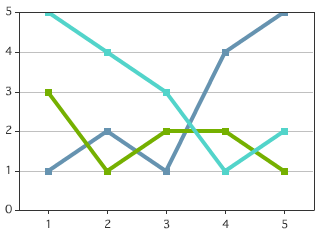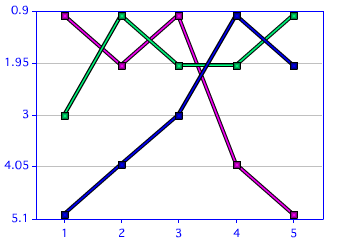Earlier yesterday morning I blogged about cfchart and a poster asked an interesting followup question:

I have another cfchart question (Maybe I am cheating by tacking it on here). I tried to chart some data based on ranked lists, like a bestseller list. I wanted to chart the position of a "book" on the list over the weeks. On the list, 1 is higher than 2 is higher than 10, so when it charted it came out upside down. I didn't see anything in the cfchart attributes that allowed me to invert this.

I have to admit, it wasn't a crucial need to chart this so I moved on to other problems, but it has sort of bugged me since then. Was I missing something easy?

His name was Magnus, and there was no way I could ignore a question from someone with a cool name like that. First let me demonstrate what he is talking about. I created some data that represented books and their ranks over a five week period: `<cfscript> function addIt(week,book,position) { queryAddRow(data); querySetCell(data, "week", arguments.week); querySetCell(data, "book", arguments.book); querySetCell(data, "position", arguments.position);} data = queryNew("week,book,position","integer,varchar,integer");addIt(1,"Alpha",1); addIt(2,"Alpha",2); addIt(3,"Alpha",1); addIt(4,"Alpha",4); addIt(5,"Alpha",5);addIt(1,"Beta",3); addIt(2,"Beta",1); addIt(3,"Beta",2); addIt(4,"Beta",2); addIt(5,"Beta",1);addIt(1,"Gamma",5); addIt(2,"Gamma",4); addIt(3,"Gamma",3); addIt(4,"Gamma",1); addIt(5,"Gamma",2); </cfscript>`

The UDF is there simply to save me some typing. I then created the chart:

`<cfchart show3d="false" > <cfloop index="book" list="#valueList(data.book)#"> <cfquery name="weekdata" dbtype="query"> select * from data where book = <cfqueryparam cfsqltype="cf_sql_varchar" value="#book#"> order by week </cfquery> <cfchartseries type="line" query="weekdata" itemcolumn="week" valuecolumn="position" serieslabel="#book#"> </cfloop> </cfchart>`

The result is a bit hard to read in static JPG format, but has nice roll overs in Flash. When I used a legend, it got a bit screwy. I'll come back to that. But as you can see, the grid starts with lower numbers first:Once again I turned to the handy dandy chart editor. After a bit of clicking I finally found what I wanted under YAxis (duh). Clicking on this setting gives you a lot of options for the YAxis, including a "Is Reversed" tick. The resulting XML gives you:

`<frameChart is3D="false"> <yAxis isReversed="true"> </yAxis> <legend isVisible="false" showColumnLegend="false"> <decoration style="None"/> </legend> </frameChart>`

Note - I did tweak a bit more, like turning off the column legend which was turned back on by the XML. This gives you the result you want - kinda:As you can see, we still have a range of 1-5. I tried like heck to fix this. There is even a range option in the chart editor, but the data seems to override this. You can supply a gridlines of 5. That does give you the right number of lines, but check out the result:Not what I'd call optimal, but I'll take a look at this a bit more later to see if I can figure this last step out. Here is the complete code of the latest version:

`<cfscript> function addIt(week,book,position) { queryAddRow(data); querySetCell(data, "week", arguments.week); querySetCell(data, "book", arguments.book); querySetCell(data, "position", arguments.position);} data = queryNew("week,book,position","integer,varchar,integer");addIt(1,"Alpha",1); addIt(2,"Alpha",2); addIt(3,"Alpha",1); addIt(4,"Alpha",4); addIt(5,"Alpha",5);addIt(1,"Beta",3); addIt(2,"Beta",1); addIt(3,"Beta",2); addIt(4,"Beta",2); addIt(5,"Beta",1);addIt(1,"Gamma",5); addIt(2,"Gamma",4); addIt(3,"Gamma",3); addIt(4,"Gamma",1); addIt(5,"Gamma",2); </cfscript><cfsavecontent variable="style"> <frameChart is3D="false"> <yAxis isReversed="true"> </yAxis> <legend isVisible="false" showColumnLegend="false"> <decoration style="None"/> </legend> </frameChart> </cfsavecontent><cfchart show3d="false" style="#style#" gridlines="5"> <cfloop index="book" list="#valueList(data.book)#"> <cfquery name="weekdata" dbtype="query"> select * from data where book = <cfqueryparam cfsqltype="cf_sql_varchar" value="#book#"> order by week </cfquery> <cfchartseries type="line" query="weekdata" itemcolumn="week" valuecolumn="position" serieslabel="#book#"> </cfloop></cfchart>`